Contents >> Applied Mathematics >> Numerical Methods >> Algebraic and Transcendental Equations >> Method of chords

 Algebraic and transcendental equations - Method of chords Method of chords (method of proportional parts) Again we’ll address to the equation (1):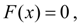where F ( x ) – is a continuous function, defined in the segment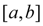and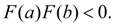There is faster way of a finding of the isolated root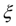of equation (1), lying in thesegment. Let's assume for definiteness, that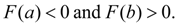Instead of thesegment halving, we’ll divide it relation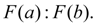It gives the first approach of a root of the equation (1):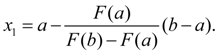(12) Then we consider the segments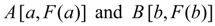. Let's choose that from them, on which ends the function F ( x ) has different signs, then we’ll receive the second approach of a root of the equation (1)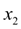and so on until then yet we’ll not reach performance of an inequality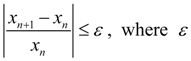– the given accuracy of the decision. Geometrically this method is equivalent to exchanging the curve у = F ( x ) with a chord, lead at first through the points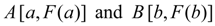and then with chords, lead through the ends of received segments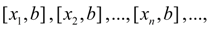(Fig. 2). From here the name – a method of chords .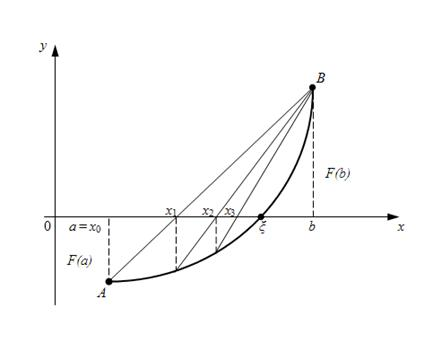Fig.2. Geometrical representation of a method of chords.

 < Previous Contents Next >